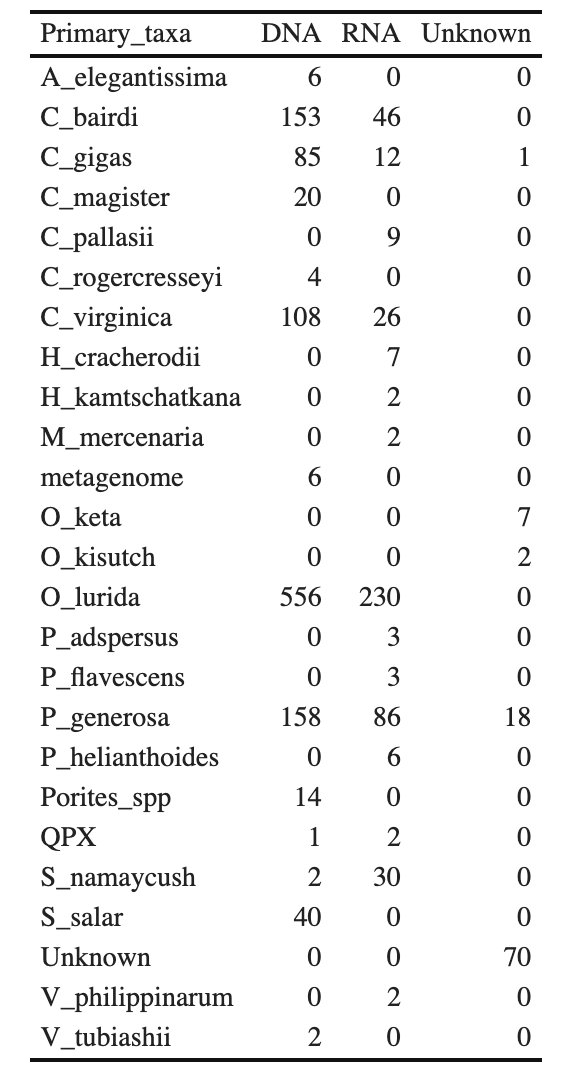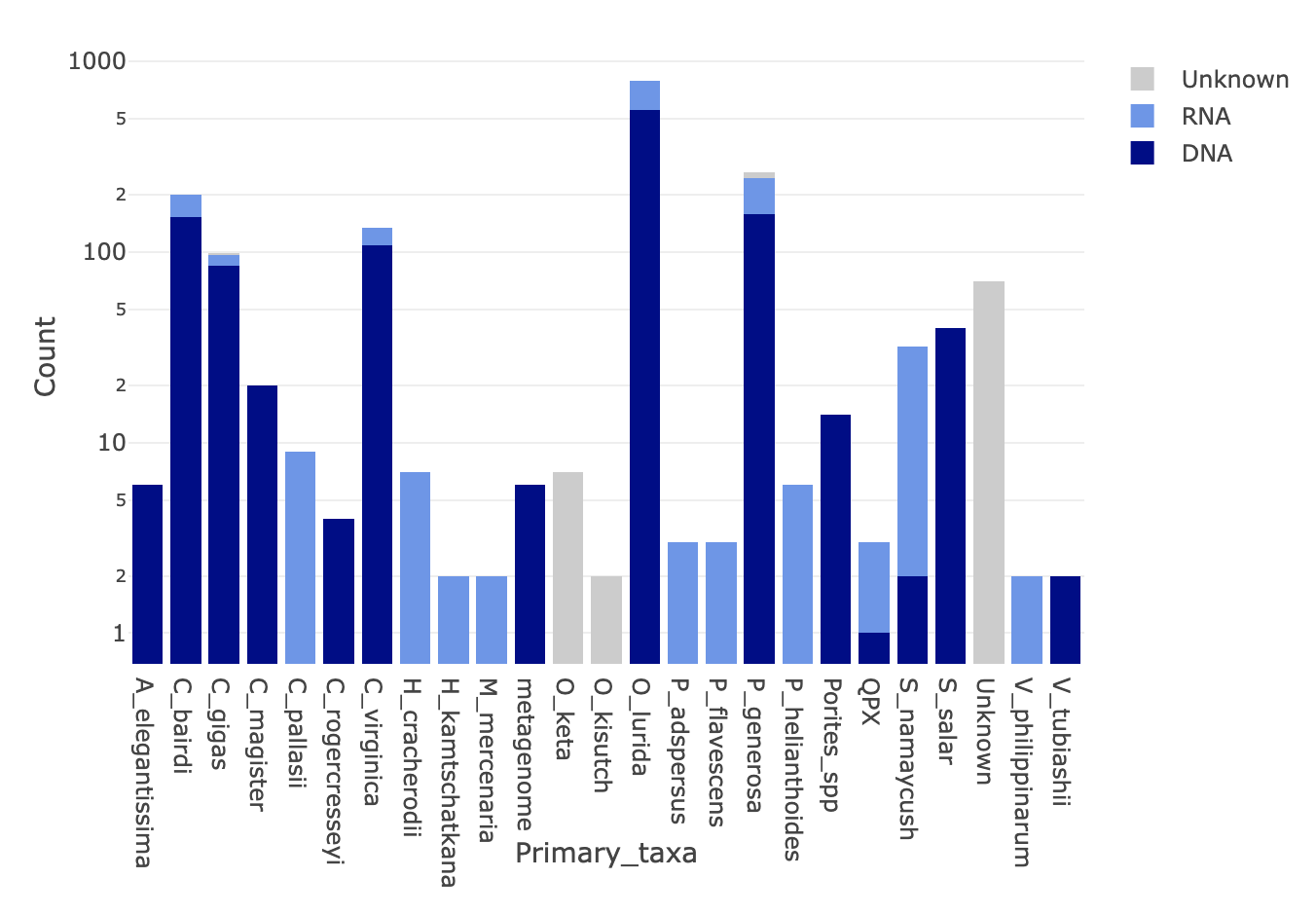# Nightingales metadata code

I created a table and figure to represent the data in the Nightingales datasheet. I have included the code so that the figure and table can be updated in the lab notebook when data is added to Nighingales.

Installing necessary packages

``````library(tidyr)
library(dplyr)
library(plotly)
library(kableExtra)
``````

Reading in data, replacing blanks and NAs

``````nightingales <- read_sheet("https://docs.google.com/spreadsheets/d/1_XqIOPVHSBVGscnjzDSWUeRL7HUHXfaHxVzec-I-8Xk/edit?usp=sharing")

nightingales[nightingales==""]<-NA

nightingales\$Primary_taxa[is.na(nightingales\$Primary_taxa)] <- "Unknown"
nightingales\$Molecule[is.na(nightingales\$Molecule)] <-"Unknown"
``````

Nightingales Data Table

``````nighttable <- (nightingales %>%
group_by(Primary_taxa, Molecule) %>% summarize(n = n(), .groups = "keep"))

options(knitr.kable.NA = '0')

nighttable %>% pivot_wider(names_from = Molecule, values_from = n) %>% kbl(digits=2) %>%
kable_classic(full_width = F, html_font = "Cambria")
``````Nightingales Figure (log y axis)

``````nighttable2 <-(nightingales %>%
group_by(Primary_taxa, Molecule) %>% summarize(n = n(), .groups = "keep")  %>% pivot_wider(names_from = Molecule, values_from = n))
nighttable2[is.na(nighttable2)] <- 0

plot_ly(nighttable2, x = ~Primary_taxa, y = ~DNA, name = 'DNA', type='bar', marker = list(color = c("#00008B")))%>%
add_trace(y = ~RNA, name = 'RNA',type='bar', marker = list(color = c("#6495ED"))) %>%
add_trace(y = ~Unknown, name = 'Unknown', type='bar',  marker = list(color = c("#CCCCCC"))) %>%
layout(barmode = 'stack', showlegend = TRUE, yaxis = list(type="log",title = "Count"))
``````Written on October 10, 2021